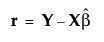Publication date: 11/10/2021

##Residual Plots

Marginal residuals reflect the prediction error based only on the fit of fixed effects. Marginal residuals are the differences between actual values and the predicted values obtained if you select Save Columns > Prediction Formula.

Denote the linear mixed model by E[Y|γ] = Xβ + Zγ. Here β is the vector of fixed effect coefficients and γ is the vector of random effect coefficients. The marginal residuals are the residuals from the fixed effects part of the predictive model:The Residual Plots option provides three visual methods to assess model fit:

Residual by Predicted Plot

Shows the residuals plotted against the predicted values of Y. You typically want to see the residual values scattered randomly about zero.

Residual Quantile Plot

Shows the quantiles of the residuals plotted against the quantiles of a standard normal distribution. Also shown is a bar chart of the residuals. If the residuals are normally distributed, the points on the normal quantile plot should approximately fall along the red diagonal line. This type of plot is also called a quantile‐quantile plot, or Q‐Q plot. The normal quantile plot also shows Lilliefors confidence bounds (Conover 1999).

Residual by Row Plot

Shows residuals plotted against row numbers. This plot can help you detect patterns that result from the row ordering of the observations.